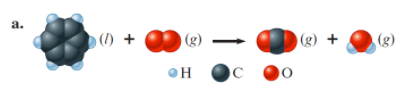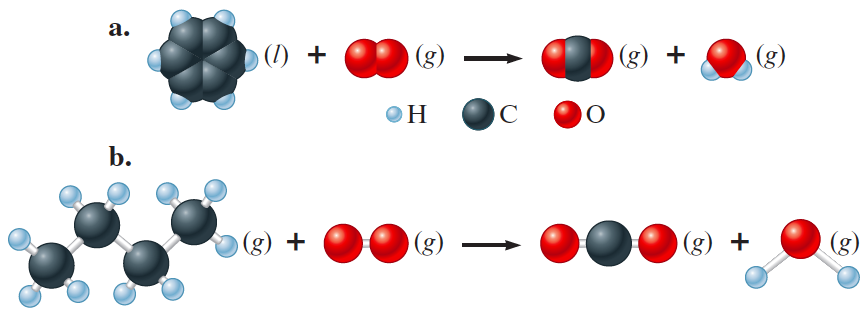# Problem: Balance the following equations representing combustion reactions:

###### FREE Expert Solution

We are being asked to balance the following equations representing combustion reactions:

Part AC6H6 (l) + O2 → CO2 (g) + H2O (g)

Reactant       Product

C         6                  1

H         6                  2

O         2                   3

81% (66 ratings)###### Problem Details

Balance the following equations representing combustion reactions:Frequently Asked Questions

What scientific concept do you need to know in order to solve this problem?

Our tutors have indicated that to solve this problem you will need to apply the Balancing Chemical Equations concept. You can view video lessons to learn Balancing Chemical Equations. Or if you need more Balancing Chemical Equations practice, you can also practice Balancing Chemical Equations practice problems.

What professor is this problem relevant for?

Based on our data, we think this problem is relevant for Professor Castaneda's class at UMICH.

What textbook is this problem found in?

Our data indicates that this problem or a close variation was asked in Chemistry: An Atoms First Approach - Zumdahl Atoms 1st 2nd Edition. You can also practice Chemistry: An Atoms First Approach - Zumdahl Atoms 1st 2nd Edition practice problems.